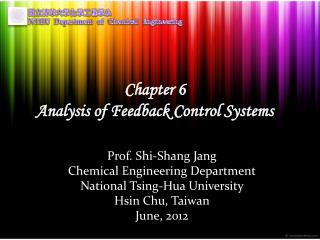DownloadDownload PresentationChapter 6 Analysis of Feedback Control Systems

# Chapter 6 Analysis of Feedback Control Systems

Download Presentation## Chapter 6 Analysis of Feedback Control Systems

- - - - - - - - - - - - - - - - - - - - - - - - - - - E N D - - - - - - - - - - - - - - - - - - - - - - - - - - -
##### Presentation Transcript

1. Chapter 6Analysis of Feedback Control Systems Prof. Shi-Shang Jang Chemical Engineering Department National Tsing-Hua University Hsin Chu, Taiwan June, 2012

2. 6-1 Introduction • Given a plant with a transfer function of y(s)/m(s)=Gp(s), a controller is implemented with a transfer function of Gc(s), the system hence becomes a closed loop function. • It is very convenient to analyze the closed loop system in s-domain since only algebraic equations are involved. • In a closed loop system, there are two inputs for the system, the set point (servo) of the output and the disturbances (regulation) that affect the output.

3. 6-1 Introduction – Cont. Variations in outlet temperature are sensed by the sensor-transmitter and sent to the controller causing the controller output signal to vary. This is in turn causes the control valve position and consequently the steam flow To vary. The variations in steam flow cause the outlet temperature to vary, thus completing the loop.

4. Example: The Heat Exchanger System

5. 6-1.1 Closed-Loop Transfer Function

6. 6-1.1 Closed-Loop Transfer Function - Continued Z=Output Zi=Input f=product in forward path from Zi to Z l=product of every transfer function in feedback loop

7. 6-1.1 Closed-Loop Transfer Function - Continued

8. Example

9. Example – Cont.

10. Example – Cont.

11. Example – Cont.

12. Example – Cont.

13. Example – Cont.

14. 6-1.1 Closed-Loop Transfer Function - Continued

15. Example – Cont.

16. 6.1.3 Steady State of Closed Loop Systems

17. Example

18. Example: New steady state of the heating tank 1. Servo control with Kc=1;Tset=1 2. Regulation Control with F=1

19. Example: Flow rate control system

20. Example: Flow rate control system – Cont.

21. 6.1.2 Characteristic Equation of the Loop

22. Example : Servo Problem for a First Order System Proportional only

23. 6.1.3 Steady State of Closed Loop Systems • Proposition 6-1：The closed loop dynamics of a first order system with a P-only controller behaves the same with another first order system, with a gain of KOL/(1+ KOL) and time constant of  /(1+ KOL) for the servo problem.

24. 6.1.3 Steady State of Closed Loop Systems– Cont. • Corollary 6-1：A P-only controller applied to a first order system can yield perfect control if Kc for a servo problem. • Proof: Consider (6-1), in case , we have K11, and 10. • Corollary 6-2：Assume that a step change with a magnitude of A at the set point Tset(s) is implemented to a P-only controller applied to a first order system, then the offset of the controller is A/(1+KOL) . • Proof: Let Tset=A/s , then To(s)=(A/s)[K1/ (1s+1)] , we have: offset =

25. 6.1.3 Steady State of Closed Loop Systems– Cont.

26. Regulation problem (disturbance rejection) using a P-only control for a first order system: 6.1.3 Steady State of Closed Loop Systems– Cont.

27. 6.1.3 Steady State of Closed Loop Systems– Cont. • Corollary 6-3：A P-only controller applied to a first order system can yield perfect control if Kc for a regulation problem. • Corollary 6-4：Assume that a step change with a magnitude of A at the load F(s) is implemented to a P-only controller applied to a first order system, then the offset of the controller is .

28. 6.1.3 Steady State of Closed Loop Systems– Cont. Kc=1 level Kc=2 Kc=5 time

29. Proposition 6-2：The offset of closed loop dynamics of a first order system with a PI controller results zero offset. Proof: 6.1.3 Steady State of Closed Loop Systems– Cont.

30. 6-2 Stability of the Control Loop

31. 6-2.1 Stability Criterion • Proposition 6-3: For a feedback control loop to be stable, all of the roots of its characteristic equation must be either negative real number or complex numbers with negative real parts. • Corollary 6-4: For a feedback control loop to be stable , all of the roots of its characteristic equation must fall on the left-hand half of the s plane, also known as the “left-hand plane” (LHP).

32. 6-2.1 Stability Criterion - Example Or Characteristic equation can be derived:

33. 6-2.1 Stability Criterion - Example

34. 6-2.1 Stability Criterion – Cont. • Consider the previous example, at Kc=0.39, the system becomes oscillatory, at Kc=60, the system becomes unstable. At this point, we term the controller gain that makes the closed loop system unstable the ultimate gain (Ku=60) of the system, the frequency (u=3.32) that the system oscillates is termed the ultimate frequency. • Problem: Given the characteristic equation C(s, Kc)=0, what is the ultimate gain and ultimate frequency of the system? • Answer: Direct substitution, Routh array, Root Locus

35. 6-2.2 Method of Direct Substitution – Cont. • Basic idea: Consider the characteristic equation C(Kc,s)=0, in case of ultimate gain, i.e. Kc=Ku, then the root of the equation should be located at the imaginary axis, i.e. s=j. The problem of solving the ultimate condition, hence changed into C(Kc,s)=R(Kc, )+jI(Kc, )=0, i.e. R(Kc, )=0, I(Kc, )=0 (two unkowns, two equations) • Problem: many algebraic work invloved.

36. 6-2.2 Method of Direct Substitution – Cont.

37. 6-2.2 Method of Direct Substitution – Cont.

38. 6-2.3 Effect of Parameters

39. 6-2.3 Effect of Parameters – Cont.